# TL;DR InfluxDB Tech Tips: Joins

If you’re an InfluxDB user you’ve almost certainly used the join() function. The join() function performs an inner join of two table streams. It’s most commonly used to perform math across measurements. However, now it is deprecated in favor of the join.inner() function which is part of the new join package. With the addition of the join package, Flux now has the ability to perform the following types of joins: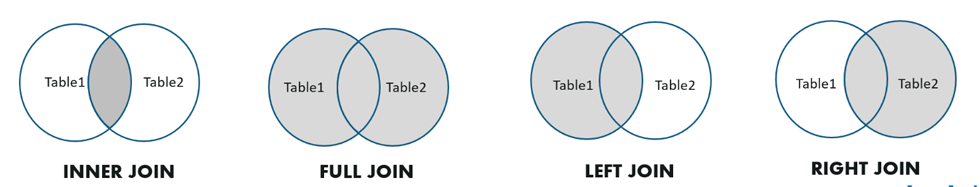In this TL;DR we’ll go over the syntax for performing different types of joins. Then we’ll answer questions provided by the community that previously had no solution because of the lack of multiple join type support in Flux.

I highly recommend reading this post in addition to this one for a complete understanding of the joins package in Flux.

## Join syntax in Flux

The joins in Flux are extremely powerful because you can create conditions and custom functions for performing different types of joins based on multiple columns. We’ll be performing the joins on the following data constructed with the array.from() function.

``````left = array.from(rows: [
{_time: 2022-01-01, label: "a", _value: 1},
{_time: 2022-01-02, label: "b", _value: 2},
{_time: 2022-01-03, label: "d", _value: 3}
])

right = array.from(rows: [
{_time: 2022-01-01, id: "a", _value: 0.4, foo: "bar"},
{_time: 2022-01-02, id: "c", _value: 0.5, foo: "bar"},
{_time: 2022-01-03, id: "d", _value: 0.6, foo: "bar"}
])``````

### Left Join

Let’s look at an example of a left join to familiarize ourselves with the syntax and the power of joins in Flux.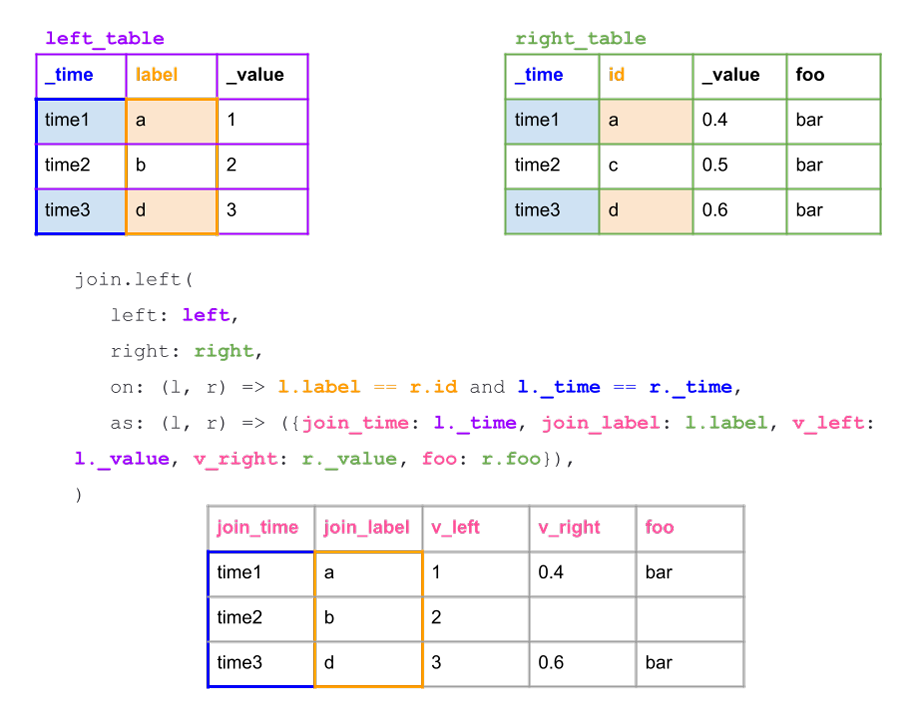For this join we assume we have queried for two table streams and named those streams `left_table` and `right_table`. The `left` and `right` parameters of the join function specify the left and right input streams respectively. Each of those tables has a `_time` column that contains the same timestamps. The left table also has a `label` column and the right table has an id column. We want to perform a left join when the following two conditions are met:

1. the values in `_time` columns match across the two tables
2. the values in the `label` and id column match across the two tables.

These conditions are specified by the `on` parameter in the join function. The `on` parameter must be a single boolean expression that compares the properties of the left and right table streams.

Finally we specify what we want returned as a part of our join with the `as` parameter. The as parameter is a user-defined function that must take a left and right stream and return a record. We decide we want to include the following columns:

1. `join_time` column: the `_time` column from the left table stream.
2. `join_label` column: the `label` column from the left table stream.
3. `v_left`: the `_value` column from the left table stream.
4. `v_right`: the `_value` column from the left table stream where the conditions specified by the on parameter are met.
5. `foo`: the `foo` column from the right table stream.

However, you might not want to explicitly name each column or return each column as shown in the image above. Instead, you can use the with clause:

``````join.left(
left: left_table,
right: right_table,
on: (l, r) => l._time == r._time and l.label == r.id,
as: (l, r) => ({l with v_right: r._value, foo: r.foo}),
)``````

This query would return the same table as above with slightly different column names: `_time, label, _value, v_right, foo`. The benefit of this syntax is the syntactic brevity that it provides. The negative is that you might have to rename columns if you want specific column names. Additionally, this approach becomes especially important when you’re joining grouped data. The with operator ensures that you don’t drop any columns in the group key. Overall, I recommend this approach.

### Right Join

Similarly, a right join on the `_time` column and the `label` and `id` columns would look like: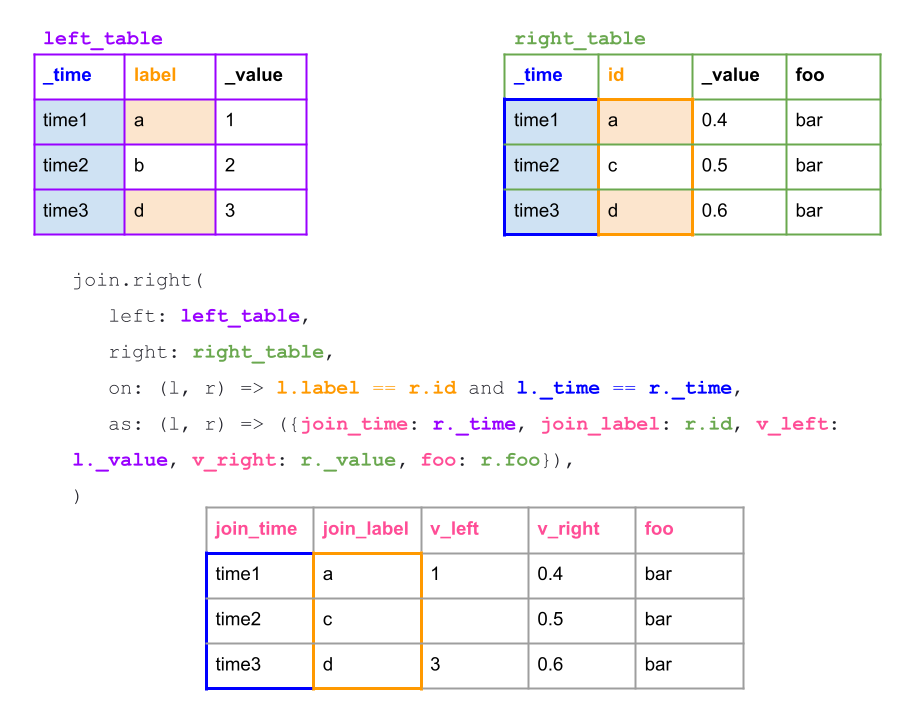Notice how the `join_label` column has changed to contain the values from the right `id` column. Similarly, the values in the `v_left`, `v_right`, and foo columns have changed to reflect the right join as well. For the `join_label` and `join_time` columns, we must pull from the right `id` column and `_time` column, respectively.

However, you might not want to explicitly name each column or return each column. Note that you can also use the with clause:

``````join.left(
left: left_table,
right: right_table,
on: (l, r) => l._time == r._time and l.label == r.id,
as: (l, r) => ({r with l_right: l._value, foo: r.foo}),
)``````

This query would return the same table as above with slightly different column names: `_time`, `label`, `_value`, `l_right`, `foo`. The benefit of this syntax is the syntactic brevity that it provides while the negative is that you might have to rename columns if you want specific column names. Additionally, this approach becomes especially important when you’re joining grouped data. The with operator ensures that you don’t drop any columns in the group key. Overall, I recommend this approach.

### Full Join

Let’s take a look at a more sophisticated example of defining a function `as` a part of the as parameter with a full join.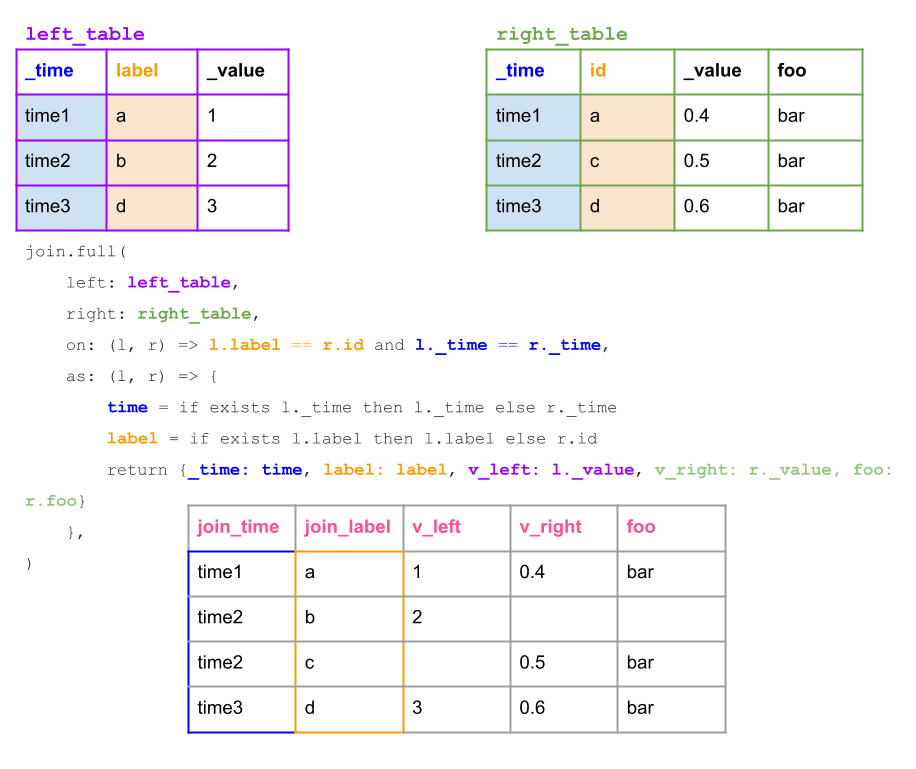We specify what we want returned as a part of our join with the as parameter. Since we want to perform a full outer join, we pull a timestamp and label values from both the right and left tables with conditional logic and the exists operator. Finally we return the columns we want with the time, label, and left and right values.

### Inner Join

Following the other join examples, let’s perform an inner join on the data on both the `_time` column and the `label` and `id` columns.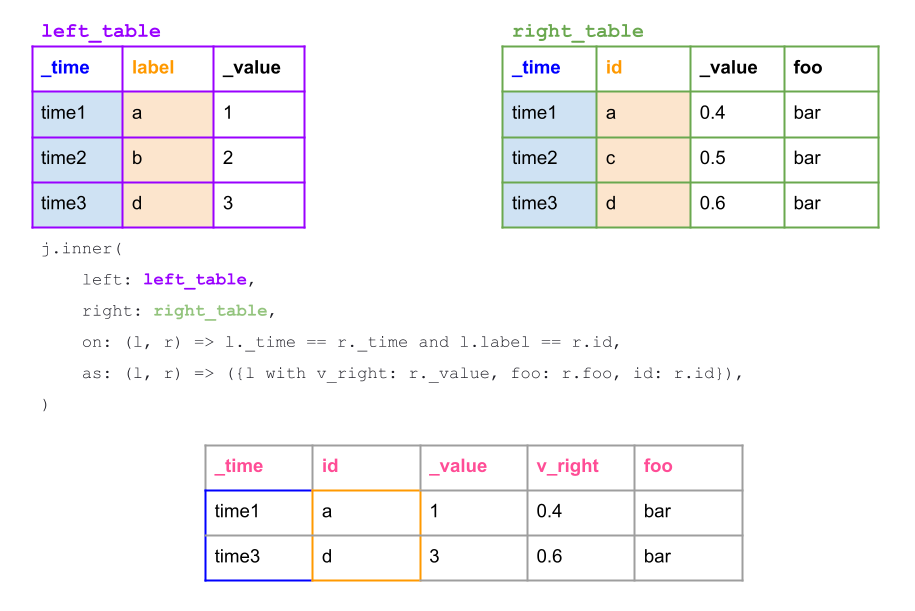The new inner join works similarly to the old join. For example, the following two queries would be equivalent:

``````// use an alias to distinguish between the universe join function and the functions that are a part of the join package
import j "join"
// new join
j.inner(
left: left_table,
right: right_table,
on: (l, r) => l._time == r._time,
as: (l, r) => ({l with v_right: r._value, foo: r.foo, id: r.id}),
)
|> yield(name: "new")
// deprecated join (the table output is identical aside from different column names)
join(tables: {left_table: left_table, left_table: right_table}, on: ["_time"])
|> yield(name: "deprecated")``````

Yielding the following table:

 _time _value v_right foo id label time1 1 0.4 bar a a time2 2 0.5 bar c b time3 3 0.6 bar d d

However, a powerful component of the new join package is the ability to perform joins on multiple columns as demonstrated previously. By contrast, if you tried to join on both the `_time` column and the `_id` column, you wouldn’t be able to return a result. In other words, the following query wouldn’t return a result:

``join(tables: {left_table: left_table, left_table: right_table}, on: ["_time","id"])``

This does bring up an interesting use case. Users might not always want to join on multiple columns. To simplify the syntax for this use case, the Flux team created the join.time() function to join on the `_time` column exclusively.

### Join on Time

The join.time() function always joins tables exclusively on the `_time` column. This way you don’t have to provide a value for the `on` parameter. Instead you just provide the join `method` type and the function for the `as` parameter. For example, the following join functions would be equivalent:

``````// use an alias to distinguish between the universe join function and the functions that are a part of the join package
import j "join"
// inner join on _time
j.inner(
left: left_table,
right: right_table,
on: (l, r) => l._time == r._time,
as: (l, r) => ({l with v_right: r._value, foo: r.foo, id: r.id}),
)
|> yield(name: "inner join on _time")
// time join
j.time(left: left_table, right: right_table, as: (l, r) => ({l with v_right: r._value, foo: r.foo, id: r.id}), method: "inner")
// deprecated join (the table output is identical aside from different column names)
join(tables: {left_table: left_table, left_table: right_table}, on: ["_time"])
|> yield(name: "deprecated")``````

This result is the same as the table directly above:

 _time _value v_right foo id label time1 1 0.4 bar a a time2 2 0.5 bar c b time3 3 0.6 bar d d

## Example with null column injection and conditionally joining null values

Part of the power of the join package is the ability to perform multiple different joins on multiple columns. Equally as important is the ability to specify your own custom as function and the ability to make that as complex as you want. You can even anticipate changes in the series you’re trying to join together to accommodate for instances where you have missing columns. For example, imagine we want to regularly join data as a part of a task. However the presence of one column from one table is sparse. We can use a combination of null value injection and conditional logic to still perform a join. This solution is to circumnavigate an error like `runtime error @31:12-39:3: left: table is missing label "column name"`. Let’s take a look at the following example:

`table1` has three columns where columns foo and bar have some null values:

 on foo bar 1 1 2 3

Notice how `table2` has an additional column `yeet` which `table1` is missing:

 on foo bar yeet 1 0 4 42 2 2 5 42

Imagine we want to perform a left join. We need to use conditional logic as a part of the function parameter to specify what we want to return in the instances where we have null values in the foo and bar column of table1. We also need to use the debug.null() function in a map() function to create an empty column with null values so that the join doesn’t fail.

 on foo bar yeet 1 1 4 42 2 2 3 42

Try this out for yourself with the following Flux code:

``````import "experimental/array"
import "array"
import "join"
import "internal/debug"

table1 = array.from(rows: [{pivot: 1, baz: "foo"}, {pivot: 3, baz: "bar"}])
|> pivot(rowKey:["pivot"], columnKey: ["baz"], valueColumn: "pivot")
|> map(fn: (r) => ({ r with on: 1 }))
|> cumulativeSum(columns: ["on"])
// ignore the code before this line, this is just to create a good example.
// use the debug.null function to create a new column with null values so the join doesn’t fail.
|> map(fn: (r) => ({ r with  yeet: debug.null(type: "string") }))
|> yield(name: "table1")

table2 = array.from(rows: [{on: 1, foo: 0, bar: 4, yeet: "42"}, {on: 2, foo: 2, bar: 5, yeet: "42"}])
|> yield(name: "table2")

joined = join.left(left: table1, right: table2, on: (l, r) => l.on == r.on, as: (l, r) => {
return {
foo: if exists l.foo then int(v: l.foo) else int(v: r.bar),
yeet: if exists l.yeet then int(v: l.yeet) else int(v: r.yeet),
bar: if exists l.bar then int(v: l.bar) else int(v: r.bar),
}
})

joined |> yield(name: "joined")``````

## Solving old problems with the new Join package

Now that we understand the syntax of the new Join package, let’s answer some old questions that couldn’t be solved without the addition of new join types.

### Problem and Solution 1

The user wanted to compare different field values across two tag keys at different timestamps. They had one field, price, and two tag values, Bookie 1 and Bookie 2. This data is displayed in the first table. However, please note that the user pivoted the field key and field value to more easily represent their data (as if the schema.fieldsAsCol() function had already been applied). Next they want to pivot their data to get the second table. Finally they want to filter for their data and perform an outer join. Backfilling data gives the user the desired result in the third table.

 _time Price Bookmaker 18:50 20 Bookie1 18:55 18 Bookie2 19:00 21 Bookie1 19:05 20 Bookie2 _time Bookie1 Bookie2 18:50 20 18:55 18 19:00 21 19:05 20 _time Bookie1 Bookie2 18:50 20 0 18:55 20 18 19:00 21 18 19:05 21 20

Let’s take a look at the Flux script that we could use to achieve this:

``````import "array"
import "join"

//create the data. We use arbitrary timestamps.
data =
array.from(
rows: [
{_time: 2022-01-01T00:00:00Z, Price: 20, Bookmaker: "Bookie1"},
{_time: 2022-02-01T00:00:00Z, Price: 18, Bookmaker: "Bookie2"},
{_time: 2022-03-01T00:00:00Z, Price: 21, Bookmaker: "Bookie1"},
{_time: 2022-04-01T00:00:00Z, Price: 20, Bookmaker: "Bookie2"},
],
)

// Pivot the data to get the second table
data
|> pivot(rowKey:["_time"], columnKey: ["Bookmaker"], valueColumn: "Price")
|> fill(column: "Bookie1", usePrevious: true)
|> fill(column: "Bookie2", usePrevious: true)
|> yield(name: "solution before joins")

// filter for the right values to prepare for the join. This filter could also be performed before the pivot and we could pivot for each Bookmaker tag value.

left = data
|> filter(fn: (r) => r.Bookmaker == "Bookie1")

right = data
|> filter(fn: (r) => r.Bookmaker == "Bookie2")

// join the left and right tables together and fill the missing timestamps.
join.full(
left: left,
right: right,
on: (l, r) => l._time == r._time,
as: (l, r) => {
time = if exists l._time then l._time else r._time
return {_time: time, Bookie1: l.Price, Bookie2: r.Price}
},
)
|> fill(column: "Bookie1", usePrevious: true)
|> fill(column: "Bookie2", usePrevious: true)
|> yield(name: "solution after joins")``````

However, part of what makes Flux so powerful is that you can solve problems in multiple ways. You don’t have to solve this problem with joins. You can instead use the debug.null() function to perform the following query to solve Problem 1:

``````import "array"
import "internal/debug"

data =
array.from(
rows: [
{_time: 2022-01-01T00:00:00Z, Price: 20, Bookmaker: "Bookie1"},
{_time: 2022-02-01T00:00:00Z, Price: 18, Bookmaker: "Bookie2"},
{_time: 2022-03-01T00:00:00Z, Price: 21, Bookmaker: "Bookie1"},
{_time: 2022-04-01T00:00:00Z, Price: 20, Bookmaker: "Bookie2"},
],
)
data
|> map(
fn: (r) => {
bookie1Value = if r.Bookmaker == "Bookie1" then r.Price else debug.null(type: "int")
bookie2Value = if r.Bookmaker == "Bookie2" then r.Price else debug.null(type: "int")

return {_time: r._time, bookie1: bookie1Value, bookie2: bookie2Value}
},
)
|> fill(column: "bookie1", usePrevious: true)
|> fill(column: "bookie2", usePrevious: true)``````

This solution is the preferred solution, but the previous solution helps us to understand how joins work and highlights the versatility of Flux.

### Problem and Solution 2

This user had an event tag. They wanted to filter for data that was written between the time that event occurred and 10 seconds after for every time that an event was recorded.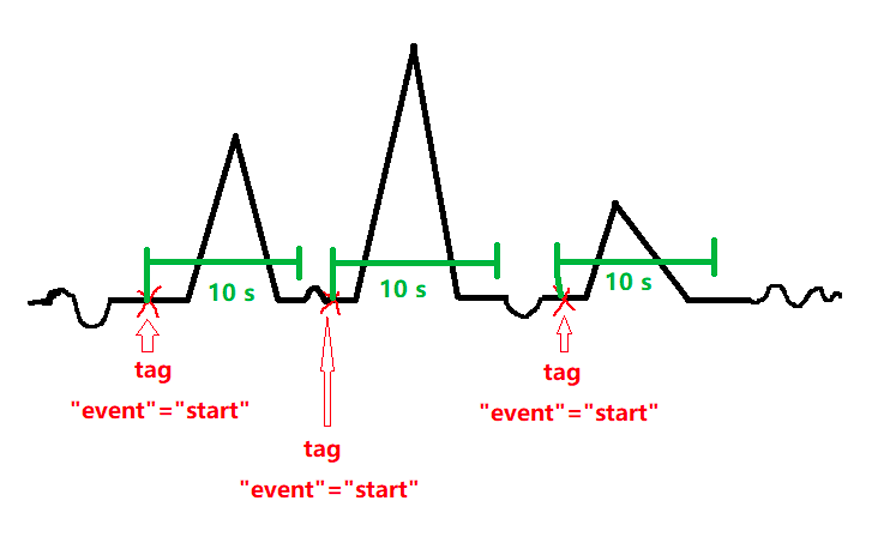``````import "array"
import "join"
import "experimental"

// our event data or StartData
startData =
array.from(
rows: [{_time: 2022-01-01T00:00:00Z, _value: 2, event: "start"},
{_time: 2022-01-01T00:00:15Z, _value: 2, event: "start"}
]
)
// we add a stop column, “myStop” with a timestamp 10 seconds after each event timestamp.
|> map(fn: (r) => ({ r with myStart: r._time }))
|> map(fn: (r) => ({ r with myStop: experimental.addDuration(d: 10s, to: r._time) }))

// the data we want to filter for based on the startData start and stop times. In this example we expect to filter out all the data where the timestamps are outside of 0-10s and 15-25s, or where the value is equal to 0.
data =
array.from(
rows: [
{_time: 2022-01-01T00:00:03Z, _value: 1},
{_time: 2022-01-01T00:00:05Z, _value: 1},
{_time: 2022-01-01T00:00:08Z, _value: 1},
{_time: 2022-01-01T00:00:12Z, _value: 0},
{_time: 2022-01-01T00:00:17Z, _value: 1},
{_time: 2022-01-01T00:00:18Z, _value: 1},
{_time: 2022-01-01T00:00:20Z, _value: 1},
{_time: 2022-01-01T00:00:23Z, _value: 1},
{_time: 2022-01-01T00:00:27Z, _value: 0},
{_time: 2022-01-01T00:00:30Z, _value: 0}
],
)

// perform a full join
join.full(
left: startData,
right: data,
on: (l, r) => l._time == r._time,
as: (l, r) => {
time = if exists l._time then l._time else r._time
value = if exists l._value then l._value else r._value

return {_time: time, value: value, myStart: l.myStart, myStop: l.myStop}
},
)
|> fill(column: "myStart", usePrevious: true)
// finally filter for data that’s 10 seconds after each event.
|> filter(fn: (r) => r._time >= r.myStart and r._time <= r.myStop)``````

Please note that this solution has some bugs. Please engage on this issue #5127 if you’re currently trying to solve this type of problem.

### Problem and Solution 3

This user wanted to identify irregular seasonality in their data.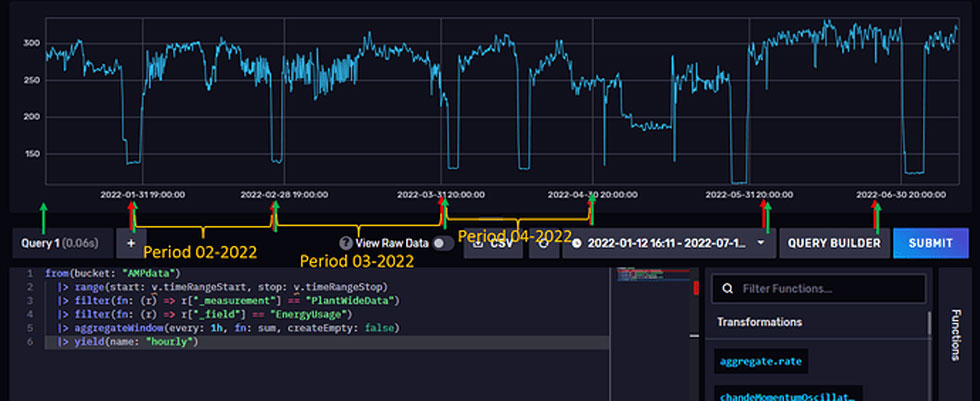To solve their problem, we’ll use the first 400 points from the NOAA sample dataset and group the data into separate periods. Here’s what the data looks like raw: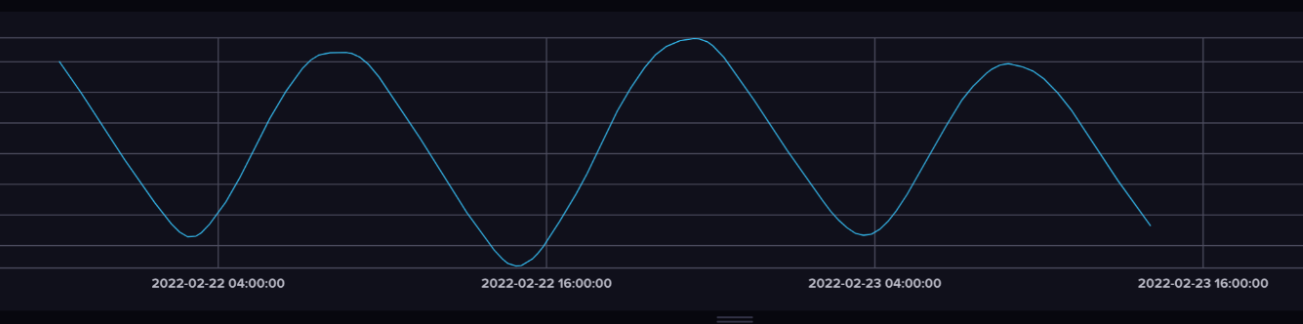Then we use Flux to group the data into periods and produce the following results: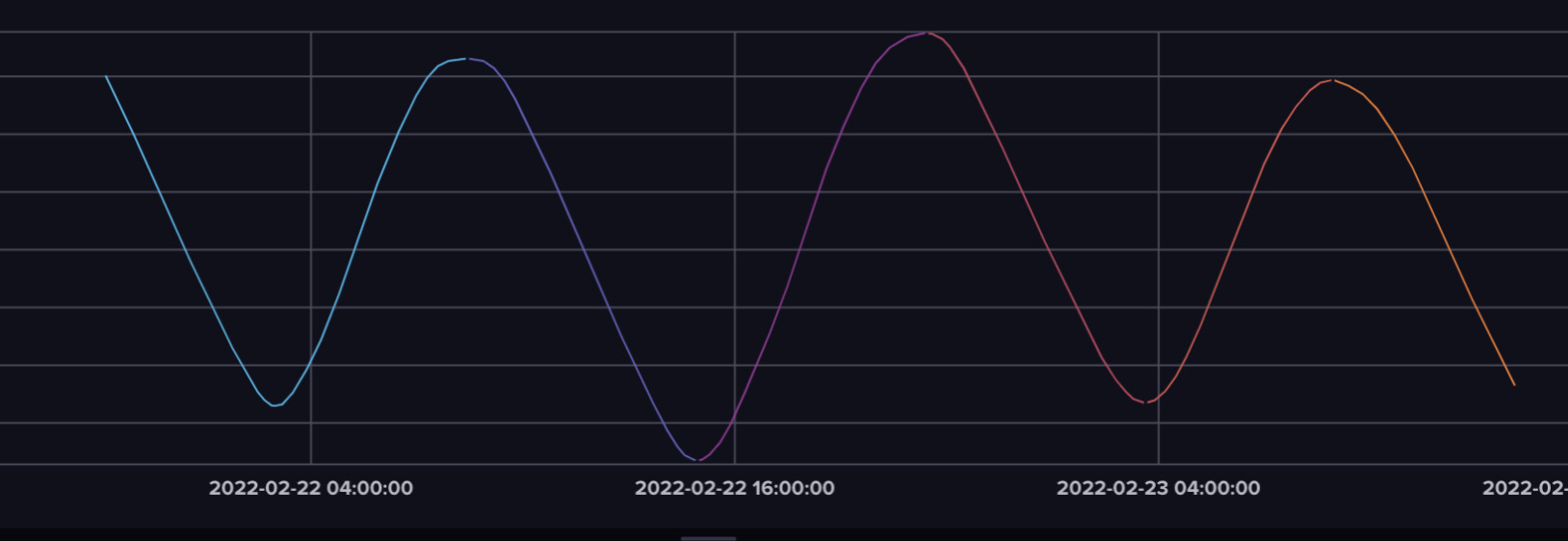Here is the general approach:

• Calculate the derivative (`der`).
• Shifting the `der` results by one timestamp (`der_shift`) and join them together `local_min_max`.
• Filter for when a change in slope sign happens.
• Use the elapse() function to account for when the derivative actually equals 0, or to make sure that the change in slope sign is at a local minima or maxima.
• Add an index to those timestamps where there are slope sign changes.
• Perform an outer join and fill previous so we can determine which values in our original data are part of which “period”.
• Grouping by the label to visualize our different “periods”.
``````import "math"
import outer "join"
// data is written 6 minutes apart
data = from(bucket: "noaa")
|> range(start: v.timeRangeStart, stop: v.timeRangeStop)
|> filter(fn: (r) => r["_measurement"] == "h2o_feet")
|> filter(fn: (r) => r["_field"] == "water_level")
|> filter(fn: (r) => r["location"] == "coyote_creek")
|> keep(columns: ["_time", "_value"])
|> limit(n: 400)
//   |> yield(name: "raw")

// find the derivatives
der = data
// unit for the time in between timestamps
|> derivative(unit: 6m)
|> keep(columns: ["_time", "_value"])
// |> yield(name: "der")

// shift the derivatives by one interval
der_shift = der
|> timeShift(duration: 6m)
|> keep(columns: ["_time", "_value"])
// |> yield(name: "der_shift")

// join the derivative and shifted derivative data together to find where the derivative changes from positive to negative between the original and shifted derivatives
local_max_min = join(tables: {der: der, der_shift: der_shift} , on: ["_time"])
// filter to find where the slope changes
|> filter(fn: (r) => r._value_der >= 0.0 and r._value_der_shift <= 0.0 or r._value_der <= 0.0 and r._value_der_shift >= 0.0 )
// to account for when the derivative does == 0 and define local minima/maxima points from either side of crossing from pos to neg or neg to pos
// also helps us make sure that local maxima and minima have adequate time between them. That they’re true local maxima and minima.
|> elapsed(unit: 6m)
|> filter(fn: (r) => r.elapsed > 1)
// |> yield(name: "local maxima/minima total of 5 points here")
// use the map function to assign a numerical value to group on later after we join with the original data
|> map(fn: (r) => ({r with period_group: 1.0}))
|> cumulativeSum()
|> keep(columns: ["_time", "period_group"])
// join the local maxima and minima with the original data and join based off of the period group
outer.time(left: data, right: local_max_min, as: (l, r) => ({l with label: r.period_group}), method: "full" )
|> fill(column: "label", usePrevious: true)
|> group(columns: ["label"], mode:"by")
// |> yield(name: "_result")``````

## Conclusion

All of the questions above come from questions on the forums. Feel free to view the original discussions here:

Finally it’s also worth being aware of the following issues with the joins package:

1. #5135: join.tables() method: “full” with an empty table returns “table is missing label _value”
2. #5127: Panic with outer join and fill + usePrevious

If you run into any of those errors, I encourage you to comment on the issue to help the team better understand the problem and address it quickly.

I hope this blog post inspires you to take advantage of the new join package in Flux to handle late arriving data. If you’re looking to tackle a problem that requires joins, I encourage you to give some of these functions a try. If you can’t figure it out, please reach out using our community site or Slack channel. I’d love to hear about what you’re trying to achieve and what features you’d like the task system in InfluxDB to have. Finally, If you’re developing a cool IoT application on top of InfluxDB, we’d love to hear about it, so make sure to share it on social using #InfluxDB! Additionally, feel free to reach out to me directly in our community slack channel to share your thoughts, concerns, or questions. I’d love to get your feedback and help you with any problems you run into!